Home > AC > Chapter 11 > Lesson 11.1.3 > Problem11-34

11-34.
1. Solve each equation below. Check each solution. Homework Help ✎

1. 6 − (3 + x) = 10

2. 100(x + 3) = 200

3.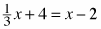4.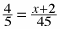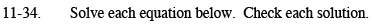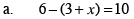Start by distributing the negative sign.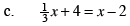Multiply both sides by 3.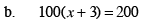Start by distributing the 100.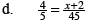Multiply both sides by 45.